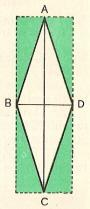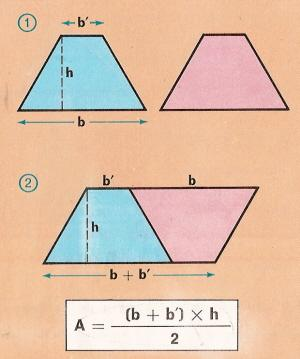Rhombus and trapezoid area to printName: ______________________________________   Subject: _______________________  Date: _______

Write the missing word

1. Area of rhombus
In the rhombus of the picture, the greater diagonal is AC and the less diagonal is BD. If we draw in the four vertices the parallels to the diagonals, we obtain a rectangle whose side is the less diagonal BD and whose height is the greater diagonal AC. If we call the diagonals d and D, the area of the rectangle will be d x D, which has 8 triangles. As the rhombus has the four interior triangles, its area will be the half of the rectangle: D x d/2.
The area of a rhombus is equal to half a product of lengths of two diagonals.

 Find the area of a rhombus whose diagonals are 3 dam and 8 m. A rhombus is D = 7 hm and d = 12 dam. How many m2 is it? Find the area of a rhombus whose diagonals are 3. 5 dam and 0.12 hm2. Area of trapezium
If we cut the red trapezoid and we put it reversed next to the blue trapezoid, we obtain a parallelogram formed by two equal trapezoids.
The width of the parallelogram is b + b ' and the height is h. Its area is (b + b') x h.
The area of the trapezoid is one-half the height times the sum of the bases, that is, (b + b') x h/2.

 Find the area of a trapezium whose bases are 6 cm and 4 cm and its height is 2 cm. The bases of a trapezium are 8 dm and 6 dm and its height is 32 cm. What is its area? Find the area of a trapezium whose height is 0.6 m and the bases are 15 dm and 1.2m.3. Exercises

 What is the area of the trapezium 1? What is the area of the rectangle 2? What is the perimeter of the rectangle 2? Find the area of the rhomboid 3. Find the area of the triangle 4.

4. Problems
 How many square floor tiles do we need to tile a room of 16 m long and 12 m wide, if the floor tiles are 20 cm side? What should be the length of a class that has 4 m wide so it can receive 44 students as a result of 1 m2 per student? Find the side of an equilateral triangle whose perimeter is 7.5 m (in m). Find the area of a square whose perimeter is equal to an equilateral triangle of 4m side (in m2) A rectangular garden is 3780 m2. What is its height if it is 42 m width? How many m2 is a rhombus whose diagonals are 12.6 m and 18.2 m? How many dam2 is a trapezium whose bases are 36 m and 48 m and its height is 25 m? The area of a rhomboid field is 37 ha, 6a and 56 ca and its altitude is 864 m. Find the meters of the base. Find the height (in m) of a rhomboid whose base is 25 dm, if its area is 4 m2. Find the side of a equilateral triangle (in dm) whose perimeter is equal to a rhombus of 3.15 dm side.

| Educational applications | Mathematics |
In Spanish  | Interactive

®Arturo Ramo García.-Record of intellectual property of Teruel (Spain) No 141, of 29-IX-1999
Plaza Playa de Aro, 3, 1ş DO 44002-TERUEL